Math resources Geometry Area

Area of a triangle

# Area of a triangle

Here you will learn about the area of a triangle, including how to find the area of a triangle with given dimensions.

Students will first learn about area of a triangle as part of geometry in 6th grade.

## What is the area of a triangle?

The area of a triangle is the amount of enclosed two-dimensional space that three connected straight lines take up.

\begin{aligned} \text { Area of a triangle } & =\cfrac{\text{ base } \times \text{ height }}{2} \\\\ & =\cfrac{1}{2} \, b h \end{aligned}

For example,

Find the area of the triangle,

\text{Area of a triangle}=\cfrac{5 \, \times \, 8}{2}=20 \, cm^2.

Your final answer must be given in square units. For example cm^2, m^2, or mm^2.

In order to find the area of a triangle, you start with the area of a rectangle and divide it by two.

The area of the rectangle below would be calculated by multiplying the base by the height (b \times h) or the length by the width (l \times w). This is pronounced as “base times height” or “length times width,” respectively.

You can draw a diagonal line through the rectangle to create two congruent right triangles.

The area of each triangle is half the area of the rectangle.

On this page, you’ll look at the area of scalene triangles. A scalene triangle is a triangle where all of the sides and all of the angles are different.

This means that a scalene triangle can contain three acute angles, a right angle, or one obtuse angle (and two acute angles).

Acute scalene triangleRight scalene triangleObtuse scalene triangle

A, \, B, \, C<90^{\circ}

C=90^{\circ}

C>90^{\circ}

### What is the area of a triangle?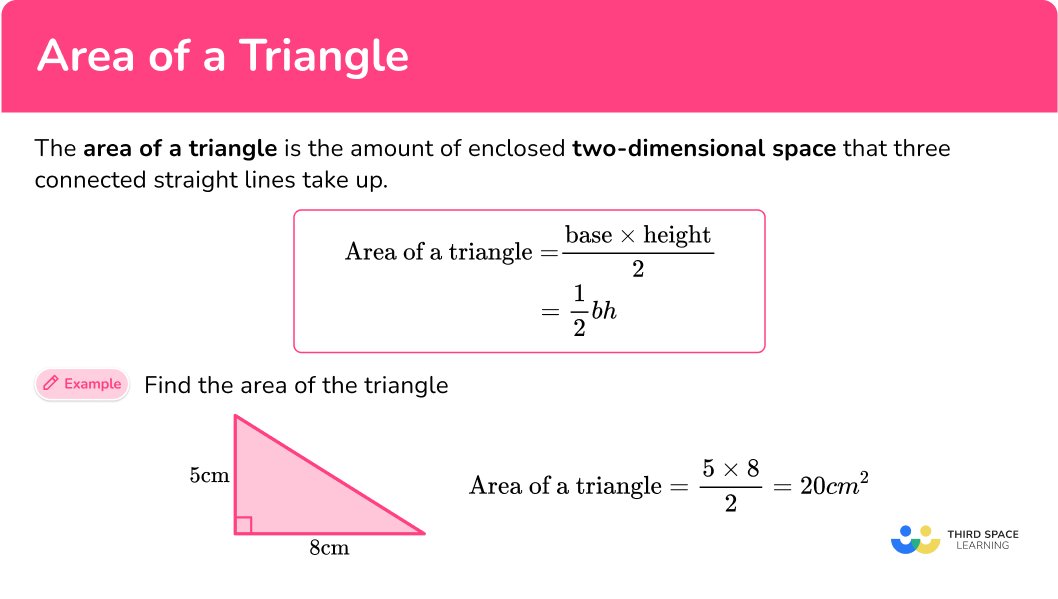## Common Core State Standards

How does this relate to 6th grade math?

• Grade 6 – Geometry (6.G.1)
Find the area of right triangles, other triangles, special quadrilaterals, and polygons by composing into rectangles or decomposing into triangles and other shapes; apply these techniques in the context of solving real-world and mathematical problems.

## How to calculate the area of a triangle

In order to calculate the area of a triangle:

1. Identify the base and perpendicular height of the triangle.
2. Write the area formula.
3. Substitute known values into the area formula.
4. Solve the equation.
5. Write the answer, including the units.

## Area of a triangle examples

### Example 1: right scalene triangle

Calculate the area of the right triangle ABC. Write your answer in square centimeters.

1. Identify the base and perpendicular height of the triangle.

The sides AC and BC are perpendicular to each other (the angle at C is a right angle), so you can state the values for the base and the perpendicular height of the triangle to be:

The base: b=3.2 \, cm

The perpendicular height: h=5.3 \, cm

2Write the area formula.

A=\cfrac{1}{2} \, bh

3Substitute known values into the area formula.

\begin{aligned} A &=\cfrac{1}{2} \, bh \\\\ &= \cfrac{1}{2} \, (3.2)(5.3) \end{aligned}

4Solve the equation.

Find the value of A by solving the equation:

\begin{aligned} A &=\cfrac{1}{2} \, bh \\\\ &= \cfrac{1}{2} \, (3.2)(5.3)\\\\ &=\cfrac{1}{2} \, \times 3.2\times 5.3\\\\ &=8.48 \end{aligned}

5Write the answer, including the units.

A=8.48\text{ cm}^2

### Example 2: obtuse scalene triangle

For triangle ABC, the angle at C is an obtuse angle, the length AC=12 \, cm, and the height of the triangle is 9 \, cm. Calculate the area of triangle ABC.

Identify the base and perpendicular height of the triangle.

Write the area formula.

Substitute known values into the area formula.

Solve the equation.

Write the answer, including the units.

### Example 3: acute scalene triangle – mixed units

Calculate the area of triangle ABC below. Write your answer in square meters.

Identify the base and perpendicular height of the triangle.

Write the area formula.

Substitute known values into the area formula.

Solve the equation.

Write the answer, including the units.

### Example 4: obtuse scalene triangle – too much information

PQR is an obtuse scalene triangle. The point S lies on the line PQ such that angle PSR=90^{\circ}. Calculate the area of the triangle PQR below to the nearest hundredth.

Identify the base and perpendicular height of the triangle.

Write the area formula.

Substitute known values into the area formula.

Solve the equation.

Write the answer, including the units.

### Example 5: compound shape

A rectangle and a triangle are placed together to make compound shape ABCDE. Calculate the area of the compound shape to the nearest tenth.

Identify the base and perpendicular height of the triangle.

Write the area formula.

Substitute known values into the area formula.

Solve the equation.

Write the answer, including the units.

## How to find a missing side length given the area

Sometimes a question might give you the area and ask you to work out the missing length. In order to do this you must rearrange the formula to make the missing value the subject, and then solve for this letter.

In order to find a missing length given the area:

1. Write the area formula.
2. Substitute the known values into the area formula.
3. Rearrange the equation and solve.
4. Write the answer, including the units.

## Missing side length example

### Example 6: calculating the base length given the area

The area of a triangle is 45 \, cm^2. The height of the triangle is 10 \, cm. Find the length of the base of the triangle.

Write the area formula.

Substitute the known values into the area formula.

Rearrange the equation and solve.

Write the answer, including the units.

### Teaching tips for area of a triangle

• To introduce the formula, give students a rectangular piece of paper and have them cut it into two equivalent right triangles. Then they can physically lay the triangles on top of each other to see that their area is equal, meaning each represents \cfrac{1}{2} of the area of the rectangle. A concrete experience such as this can help them remember the formula.

• Give students a variety of types of triangles to work with; triangles with equal sides (equilateral triangles), acute triangles, obtuse triangles, right triangles (also called right-angled triangles), and scalene triangles where the lengths of the sides are different. Show them at different angles and positions so students need to find the base of the triangle and the height of the triangle.

### Easy mistakes to make

• Incorrectly identifying the dimensions
The base of a triangle and the height of a triangle MUST be at a right angle to each other. They must be perpendicular. You may be given additional sides of a triangle that are not needed. You may also need to rotate the triangle around so that you can identify the base and height more easily.

For example,

• Forgetting to write the units
It is common to forget the units for area in the final answer. When calculating the area of a rectangle, your answer must always have units squared (or square units). This is also written by using the exponent 2 after the abbreviated units, such as unit^2.

### Practice area of a triangle questions

1. Calculate the area of the triangle: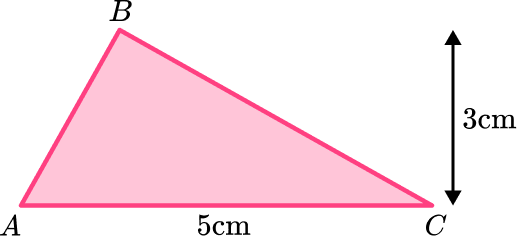8 \text{ cm}^27.5 \text{ cm}^215 \text{ cm}^216 \text{ cm}^2The base and the perpendicular height are 5 \, cm and 3 \, cm respectively, so the calculation you need to do is:

\begin{aligned} A&=\cfrac{1}{2} \, bh \\\\ &=\cfrac{1}{2} \, (5)(3)\\\\ &=\cfrac{1}{2} \, \times 5\times 3\\\\ &=7.5 \end{aligned}

The units will be cm^2.

2. Find the area of the triangle. Give your answer in cm^2 :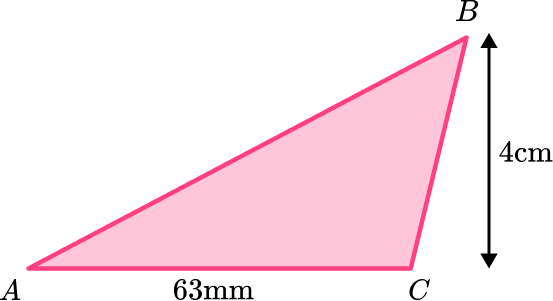126 \text{ cm}^2252 \text{ cm}^212.6 \text{ cm}^225.2 \text{ cm}^2The base is 63 \, mm which needs to be converted to 6.3 \, cm.

The height is 4 \, cm.

The calculation you need to do is:

\begin{aligned} A&=\cfrac{1}{2} \, bh \\\\ &=\cfrac{1}{2} \, (6.3)(4)\\\\ &=\cfrac{1}{2} \,\times 6.3 \times 4\\\\ &=12.6 \end{aligned}

The units will be cm^2.

3. Find the area of the triangle: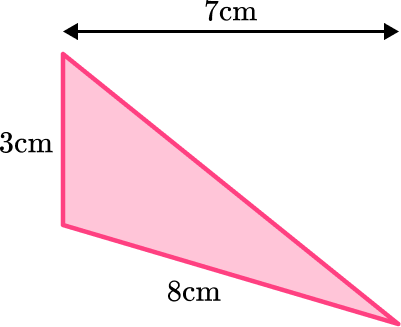24 \text{ cm}^221 \text{ cm}^212 \text{ cm}^210.5 \text{ cm}^2It may be easier to see the base and height if you turn the triangle.

The base and the height are 3 \, cm and 7 \, cm. The 8 \, cm is not needed.

So the calculation you need to do is:

\begin{aligned} A&=\cfrac{1}{2} \, bh \\\\ &=\cfrac{1}{2} \, (3)(7)\\\\ &=\cfrac{1}{2} \,\times 3 \times 7\\\\ &=10.5 \end{aligned}

The units will be cm^2.

4. Triangle ABC is an obtuse scalene triangle. The angle at A=120^{\circ}. Calculate the area of the triangle. Give your answer in m^2 :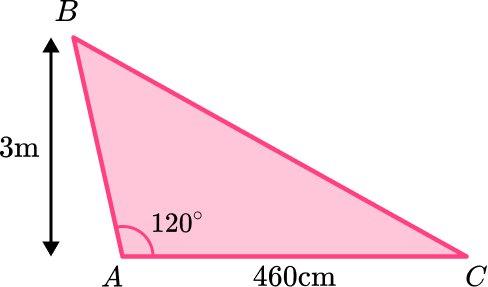13.8 \text{ m}^213 \, 800 \text{ cm}^26.9 \text{ m}^269 \, 000\text{ cm}^2The base is 460 \, cm which needs to be converted to 4.6 \, m.

The height is 3 \, m.

The calculation you need to do is:

\begin{aligned} A&=\cfrac{1}{2} \, bh \\\\ &=\cfrac{1}{2} \, (4.6)(3)\\\\ &=\cfrac{1}{2} \,\times 4.6 \times 3 \\\\ &=6.9 \end{aligned}

The units will be m^2.

5. Find the area of the compound shape. Give your answer in m^2 :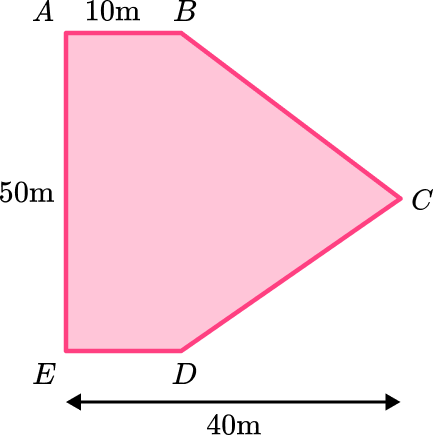1250 \text{ m}^2500 \text{ m}^2750 \text{ m}^22000 \text{ m}^2The compound shape is made up of a rectangle and a triangle.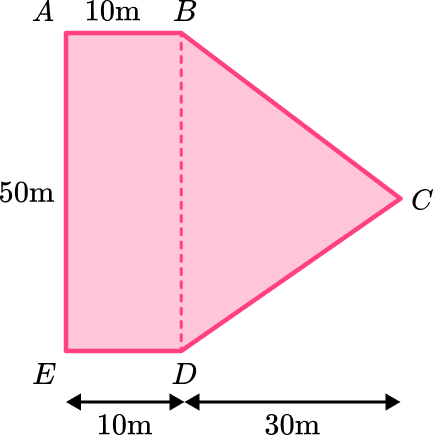The area of the rectangle is 10\times{50}=500\text{ m}^2

The area of the triangle is:

\begin{aligned} A&=\cfrac{1}{2} \, bh \\\\ &=\cfrac{1}{2} \, (50)(30) \\\\ &=\cfrac{1}{2} \,\times 50 \times 30 \\\\ &=750 \end{aligned}

The total area will be 500+750=1250

The units will be m^2.

6. The area of a triangle is 72 \, cm^2. It has a height of 12 \, cm. Find the base length of the triangle.

24 \text{ cm}12 \text{ cm}6 \text{ cm}18 \text{ cm}The area is 84 \, cm^2 and the height is 14 \, cm.

The calculation you need to do is:

\begin{aligned} A&=\cfrac{1}{2} \, bh \\\\ 84&=\cfrac{1}{2} \, (b)(14)\\\\ 84&=\cfrac{1}{2} \, \times b\times 7\\\\ b&=84\div 7\\\\ b&=12 \end{aligned}

The units will be cm.

## Area of a triangle FAQs

What is the area formula for a triangle?

The area formula for a triangle is A=\cfrac{1}{2} \, b h.

What is the difference between the area of a triangle and the perimeter of a triangle?

The area of a triangle is the amount of enclosed two-dimensional space inside the shape. The perimeter of a triangle is the length of distance around the outside of the triangle, or the added lengths of the sides of the triangle.

How does the pythagorean theorem relate to the area of a triangle?

The pythagorean theorem can be used to find a missing base or height length to then use the area formula. The pythagorean theorem can be used when the hypotenuse and either the base or height is known. Students substitute the dimensions in to find all three side lengths. Then they use the area formula using the known base and height.

## Still stuck?

At Third Space Learning, we specialize in helping teachers and school leaders to provide personalized math support for more of their students through high-quality, online one-on-one math tutoring delivered by subject experts.

Each week, our tutors support thousands of students who are at risk of not meeting their grade-level expectations, and help accelerate their progress and boost their confidence.